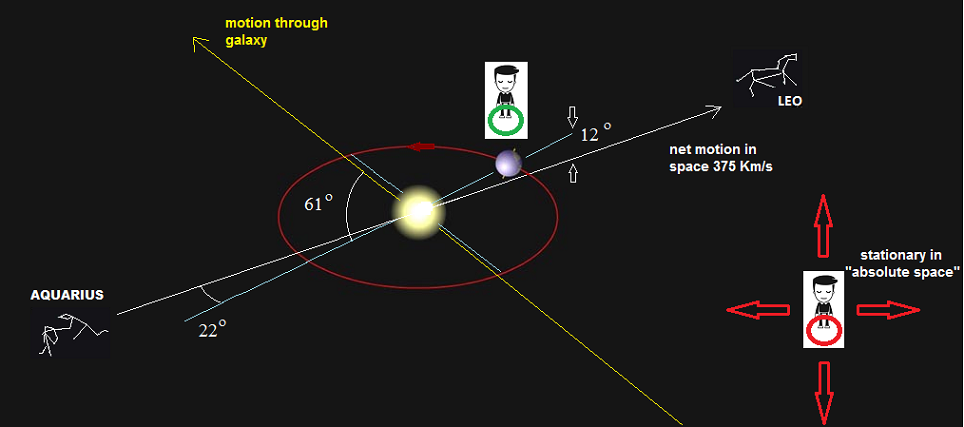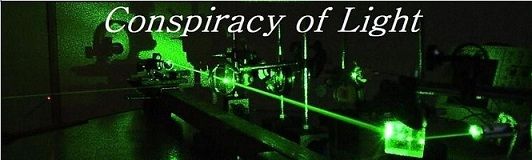The search for  new physics.

Did the Hafele and Keating Experiment Prove Einstein Wrong?

Hafele and Keating  performed an experiment in 1971 to test Einstein's predictions regarding the dilation of time in clocks moved at some velocity with respect to a stationary clock. In the opening statement of the first of two papers on the subject, the authors refer to the debate surrounding the "twins paradox" and how an experiment with macroscopic clocks might provide an empirical resolution. In Hafele and Keating's experiment, they flew cesium clocks around the world in opposite directions near the equator, and then measured how much time they have gained or lost when they return to the start point as compared to a stationary cesium clock to which they were originally synchronized. The experiment of Hafele and Keating (HK) actually differs from the twins paradox in some important ways - firstly, in the paradox, the traveling twin moves in a straight line to a distant point then turns around and comes back, with a series of accelerations and decelerations. In the HK experiment, the journey is circular and the clocks never leave the earth’s influence. In following a circular path, the HK experiment becomes a Sagnac effect experiment where it is clocks that are moving rather than beams of light.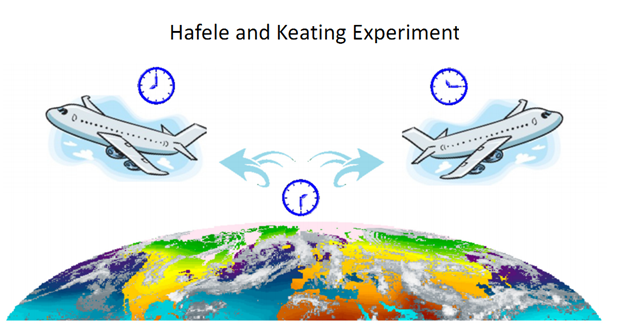From the perspective of the observer on the ground, the moving clocks of Hafele and Keating have experienced similar motions with respect to the stationary earth bound clock. If their motions were truly "relative", then by the rules of SR both should arrive back at the starting point having experienced the same amount of time dilation in the same direction. The actual result was that the westward travelled clock increased in its time rate (more time had elapsed) and the eastward travelled clock decreased in its time rate.  The  result was as follows from their 1972 paper in Table 1: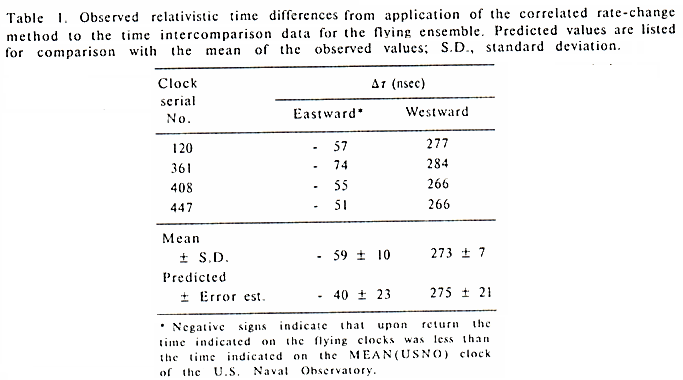The expected time dilation on clocks used by Hafele and Keating is shown below in Fig. 2. This consisted of a height term gh/c^2, a velocity time dilation term v^2/2c^2, and a third term RΩvCos(θ)/c^2 which will herein be referred to as the “Sagnac term”. The height and velocity terms were predicted by Einstein in 1907 and 1905 – however the Sagnac term was unknown to Einstein when he wrote his papers on special relativity, so this effect was never anticipated by Einstein. This effect became incorporated awkwardly into Hafele and Keating’s relativistic calculations with the following comment:

“Because the earth rotates, standard clocks distributed at rest on the surface are not suitable in this case as candidates for coordinate clocks of an inertial space. Nevertheless, the relative timekeeping behavior of terrestrial clocks can be evaluated by reference to hypothetical coordinate clocks of an underlying non-rotating (inertial) space (6). “ In note (6) they further explain: “It is important to emphasize that special relativity purports to describe certain physical phenomenon only relative to (or from the point of view of) inertial reference systems, and the speed of a clock relative to one of these systems determines its timekeeping behavior. [G. Builder, Aust. J. Physics 11, 279 (1958)]. Although inertial systems are highly specialized, they have an objective physical relationship with the universe because they have no acceleration or rotation relative to the universe. “  (my itallics added).

The clarification comes from  Builder's "Ether and Relativity" as follows:

"The relative retardation of clocks, predicted by the restricted theory of relativity, demands our recognition of the causal significance of absolute velocities. This demand is also implied by the relativistic equations of electrodynamics and even by the formulation of the restricted theory itself. The observable effects of absolute accelerations and of absolute velocities must be ascribed to interaction of bodies and physical systems with some absolute inertial system. We have no alternative but to identify this absolute system with the universe. Thus in the context of physics, absolute motion must be understood to mean motion relative to the universe, and any wider or more abstract interpretation of the "absolute" must be denied. Interactions of bodies and physical systems with the universe cannot be described in terms of Mach's hypothesis, since this is untenable. There is therefore no alternative to the ether hypothesis. "

We say “awkwardly” because in order to incorporate this Sagnac term, Hafele, Keating and Builder make some categorically un-relativistic statements, namely:

1)     Inertial motion can only be referenced to the physically privileged frame of the “universe” which is an absolute inertial system.

2)     The “absolute velocities” must mean motion with respect to this absolute frame of reference.

3)      There is no alternative to the ether hypothesis.

We will return to these issues later, but let’s continue to address the Hafele and Keating equation and apply it to low speed motions on our terrestrial sphere.  The equation is displayed below:

Fig. 2: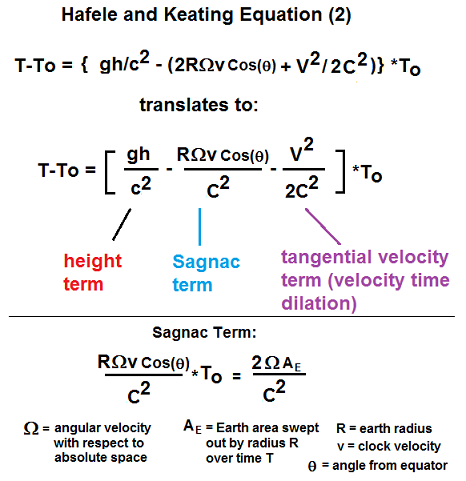Since we are calculating time dilation on a rotating sphere we also have to add a term to correct for direction, since if a plane or train goes west its velocity subtracts from earth’s rotational velocity, if it goes east it adds.  This version is shown below: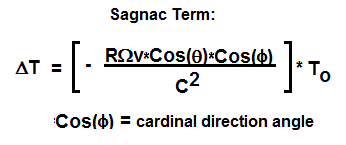Applying the Hafele and Keating equation to Einstein’s trains:

Now, if we apply this equation to the predictions Einstein’s made in his special relativity theory (SR) about time dilation in clocks on moving trains, we discover that his original predictions would appear to be in error.  We will restrict ourselves in this discussion to the case where the trains travel at the same sea level altitude, so gravitational time dilation need not be considered.

Fig. 2: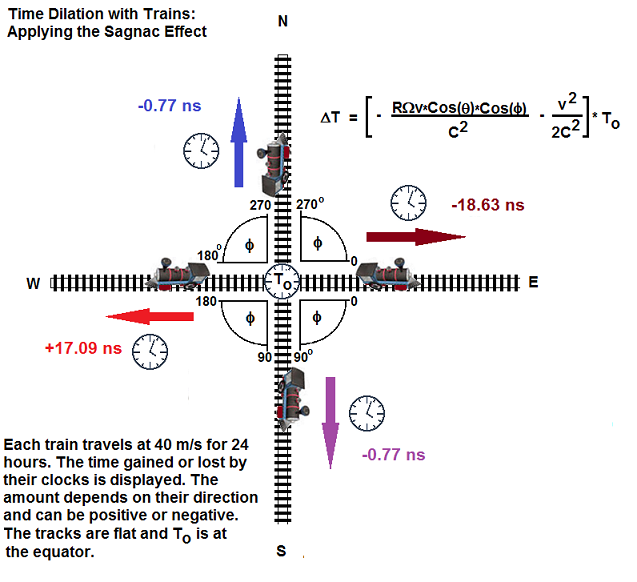As can be seen from the above figure, the total amount of time dilation experienced by each train travelling at the same speed depends on the cardinal direction.  The clock on the train travelling east counted slower compared to the stationary clock at To. The clock on the train  travelling west counter faster. The time lost on the eastbound train was 20X higher than that lost on the north and southbound trains.Einstein Prediction 1: Einstein’s assertion that for a moving clock, the time elapsing between two strokes of the clock is not one second, but 1/SQRT(1-v^2/c^2) x 1 second. Ref: A. Einstein, Relativity, the Special and General Theory, 1920, P. 44. Surprisingly, only when the train travels due North or South does Einstein’s prediction come close to being true. However, in all other directions it is off by up to 20x the predicted value. Also, time dilation according to Einstein’s restricted theory was supposed to increase with velocity – when the train travels west it actually decreases. Consequently, Einstein’s prediction ends up being false most of the time for these trains. The Sagnac effect dominates for clocks moving at the speeds normally encountered in daily life, say below 500 m/s  (1800 km/hr) in the E-W direction.

Einstein Prediction 2:  "If one of two synchronous clocks at A is moved in a closed curve with constant velocity until it returns to A, the journey lasting t seconds, then by the clock which has remained at rest the traveled clock on its arrival at A will be 1/2 t v^2/c^2 second slow. Thence we conclude that a balance-clock at the equator must go more slowly, by a very small amount, than a precisely similar clock situated at one of the poles under otherwise identical conditions. See " On the Electrodynamics of Moving Bodies 1905 p. 11.

This prediction is actually disproved by the Hafele and Keating experiment proper, since the travelled clock obeys equation (2) above. As a consequence, the travelled clock experiences either a gain or a loss of time depending on whether it travels west or east, and this amount is more than the 1/2 t v2/c2 value predicted by Einstein.

Einstein Prediction 3: “If the principle of relativity were not valid we should therefore expect that the direction of motion of the earth at any moment would enter into the laws of nature, and also that physical systems in their behavior would be dependent on the orientation in space with respect to the earth.” Ref: A. Einstein, Relativity, the Special and General Theory, 1920, P. 17-18.

As shown in Fig. 2, we can see that “the direction of motion of the earth” actually does figure in the calculation, in the form of the angular velocity of the earth W. Further, the “orientation in space with respect to the earth” also figures in the calculation, via Cos(f), since the amount of time dilation depends on whether the trains is travelling North, South, East or West along the earth.

Einstein Assertion 4: The introduction of a “luminiferous ether" will prove to be superfluous inasmuch as the view here to be developed will not require an “absolutely stationary space" provided with special properties, nor assign a velocity-vector to a point of the empty space in which electromagnetic processes take place.” See " On the Electrodynamics of Moving Bodies 1905 P.1.

Definition of a preferred frame of reference: A frame is said to be physically privileged, when there is some essential physical difference between it and the others. In most historical aether theories, the essential physical difference is that it is the only frame where the speed of light is constant.

The Hafele and Keating experiment implies that the earth’s pole, or more perfectly, the fixed stars,* compose the only frame where Einstein synchronization doesn’t break down. Here lies the problem - if you have a series of clocks around the equator of the earth and you carry a portable clock eastward to synchronize each successive clock, when you reach the last one, the first clock will lead the last clock by 207.4 ns. [ref] If Einstein synchronization is applied instead to synchronize the clocks, similar path dependent inconsistencies arise due to the rotation of the earth. Only if the clocks are synchronized to the Earth Centered Inertial (ECI) frame of reference (i.e. the pole of the earth) do these inconsistencies become manageable. However, this is tantamount to establishing the ECI frame as a preferred reference frame for motion above all others. Since the Sagnac effect is due to rotation not with respect to the earth’s pole, but more perfectly with respect to the “fixed stars”, the only true frame of reference for the Sagnac term is “absolute stationary space” which Einstein’s asserted was “superfluous.” However, Hafele and Keating must re-introduce the concept of “motion relative to the universe” to serve as an inertial frame for their hypothetical clocks – as Builder puts it – “there is therefore no alternative to the ether hypothesis." As we have pointed out in a companion article here the fixed stars compose the only frame of reference where apparent time dilation will always increase with velocity regardless of direction. Does this not make the fixed stars a physically privileged reference frame?

Einstein Assertion 5: "We will raise this conjecture (the purport of which will hereafter be called the Principle of Relativity") to the status of a postulate, and also introduce another postulate, which is only apparently irreconcilable with the former, namely, that light is always propagated in empty space with a definite velocity c which is independent of the state of motion of the emitting body." See " On the Electrodynamics of Moving Bodies 1905 P.1.

Consider the diagram in Fig. 3. We have two optical setups on a table, both perfectly stationary with respect to the laboratory observer. A beam of light is emitted in a pulse from each source and the light follows the paths shown in red, returning to the detectors where the propagation speed in calculated based on the elapsed time and the path distance. In the setup on the left, the speed of light is measured as C. For the path on the right, something more interesting happens. If we consider the mathematical treatment from Post’s seminal 1967 paper on the Sagnac effect, the time for the light beam to go around the optical path counter-clockwise (t1) or clockwise (t2) for the laboratory observer (co-rotating with the earth) is: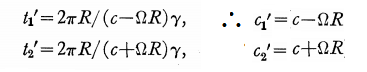A consequence of this is that the measured speed of light for the return journey of the beam from the source to the detector will never be C, but will actually be a variable speed of light, which depends on the direction the beam travels, the radius R of the light path loop and W*cos(f) of the earth similar to the situation of fig. 2.

Fig. 3: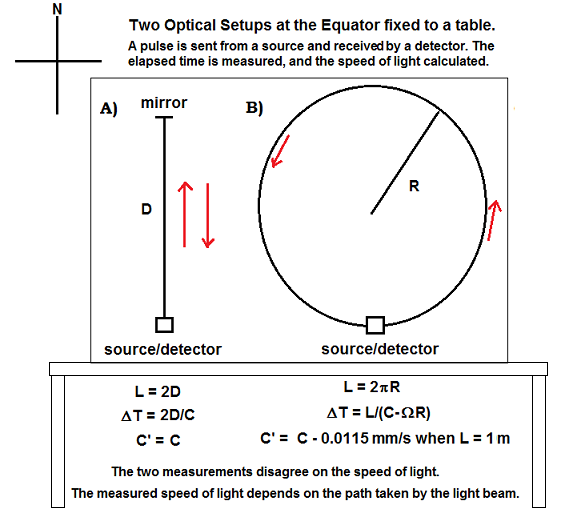For example, if we use the values above of a vertical loop of length = 1 meter and the table and observer are at the equator with a counter-clockwise beam, the speed of light is measured as C – 0.0115 mm/s. If the beam goes clockwise, the light beam will be measured to have exceeded the speed of light! We conclude from this experiment that the measured speed of light is not constant. Further, if we consider the two paths A and B to be members of a set of closed paths enclosing an area = x, then path A represents the condition where x = 0, but path B represents every other possible value of x up to infinity. So path A, where the relativistic prediction is met, is the exception, whereas generally, all other possible members of the set will be measured to have a variable speed of light C’ in the laboratory frame – which frankly is the frame that most often matters. This is similar to the situation of Fig. 2, where Einstein’s prediction is only approximately true when the train travels exactly North or South.

The argument usually goes that the setup on the right encloses an area and therefore experiences the Sagnac effect due to the rotation of the earth, and thereby the table is not an inertial frame. However, the two setups share the same frame of reference - so either both setups are invalid for SR, or both are valid. If an argument is going to be made that a speed of light test is invalid because the frame is rotating, or the observer is rotating, then we have to discard all speed of light tests to date since every place that humans have performed a test on the earth or in near space have always been experiencing some form of rotation, either diurnal or orbital, which in modern times has become readily visible.  As Hafele and Keating say in their paper, inertial motion must be referenced to a universal reference frame.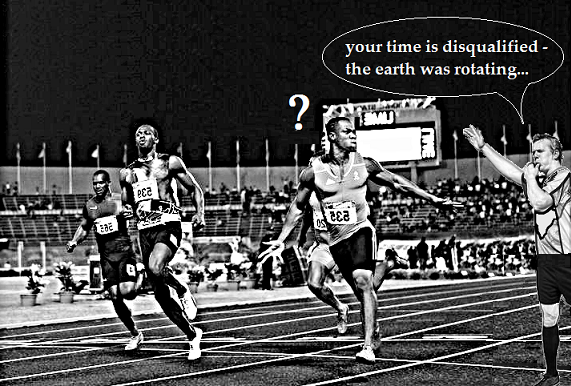Another common argument is to say that the path length has changed due to the rotation and this is why the speed of light is not measured to be C. But for our figure 3B the path length does not change for our laboratory observer since the loop is stationary in his frame. In fact, all observers anywhere on the earth would observe some degree of variable speed of light in the loop except an observer at the earth's pole** – but if we choose this singular position as the only valid observer, i.e. the only one who measures a path change sufficient that C = C’, are we not establishing the pole as a preferred frame of reference over all others? A singular frame where the speed of light is constant, just like in aether theories? Relative inertial frames of reference existed only to the extent that we could not detect their rotation now that we can routinely detect diurnal rotation in our optical instruments and clocks, can we really say that a purely inertial frame of reference still exists independent of the non-rotating universe?Fig 4: A Sphere of contradiction.

If the speed of light is constant for inertial observers, and inertial motion is referenced to an absolute inertial system, then the  speed of light is only constant in an absolute reference system or in some restricted motion with respect to it. The Hafele and Keating experiment would appear to oblige relativity to adopt the same definition as many ether theories - i.e. the speed of light is constant in a preferred reference frame similar to the ideas of Lorentz.

Conclusions

Although the Hafele and Keating experiment did confirm the gravitational time dilation effect on clocks predicted by Einstein, the velocity time dilation predictions of special relativity (1905) at low velocities would seem to have been proven wrong, since the Sagnac term was not accounted for. I personally do not consider the Sagnac effect relativistic simply because it involves time dilation - the concept of time dilation was not conceived of by Einstein, but rather by Lorentz and Larmor. Further, time dilation can be construed as simply an error in clock readings, as discussed here. The Sagnac term is at odds with a fundamental tenet of relativity theory by requiring an absolute frame of reference for both rotational and inertial motion, defining the latter as relative to a non-rotating universal frame. Why then has the Sagnac effect been rebranded as “relativistic”? Some have cited Max van Laue as discussing the effect (after the proposal of Michelson in 1904, before Einstein was even known) in terms of special relativity in 1911.  What he actually says of it is that “it's not possible to decide between the theory of relativity and the stationary aether theory by the proposed experiment.” This sentiment was continued by Silberstein, who was involved in getting Michelson to perform the Michelson-Gale Experiment (via A.C. Lunn). This is what Silberstein said about relativity and the Sagnac Effect:

”The special relativity theory is wholly incompetent to deal with the problem of light propagation in a rotating system rigorously, simply because it has nothing to do with any reference systems other than the Galilean or inertial ones, i.e. the fixed star system S*.” Silberstein further said of the general relativity treatment of the Sagnac effect: “…the relativity theory is unable to construct the required line element on its own great principles, and is content to transcribe it from the Galilean line element…”

So relativity theory would appear to contribute little to understanding the effect.  However in the mid-80’s the Hafele and Keating experiment began to be referred to as a “relativistic Sagnac experiment”, attributed to Allan, Weiss and Ashby. They said that the principle of the constancy of the speed of light leads to a prediction of the Sagnac effect in rotating systems; this is an interesting comment since the constant speed of light in their example is in the non-rotating frame of reference - i.e. the frame of the fixed stars just as in Fig. 4 above.  This underlines the extent to which "relativistic" has become interchangeable with "absolute."

Notes:

* Note – the first reference we can find mentioning that the Sagnac effect is rotation with respect to the “fixed stars” for both aether and relativity theories, is L. Silberstein’s paper from 1921.

** Even this doesn’t solve the problem, since a pole observer will still be rotating with respect to the fixed stars.

Appendix A

Redefining Motion after the Hafele and Keating Experiment

The following description would seem to be implied from the explanation of Hafele, Keating and Builder. Shown below is a drawing of our earth and sun moving through space. "Absolute space " is assumed for convenience herein to be the frame of the microwave background radiation (CMBr), although it could be some other unknown frame.  We first define our non-rotating inertial observer in stationary space, who is stationary with the CMBr. This is the observer shown below holding the red loop.  The red arrows represent "absolute velocities" (as defined by Builder) in straight lines with respect to this frame of reference.  This observer is the one referred to as an "inertial observer".  If he measures the speed of light around his loop it will be equal to C. If he sends clocks around the loop, their rate of time will be slower in either direction. This should also be true if he moves in a straight line from his stationary position at some absolute velocity.  However, this observer is unrealistic - a realistic observer would be on a planet or in space inside a solar system.  Let's place such an observer on the earth (shown with the green loop). This observer is non-inertial since he is experiencing three different rotations with respect to the CMBr - diurnal, solar, and galactic. Because of this, no matter how he orients his loop, the measured speed of light around it will never be exactly C, and will differ in each direction.  His clocks moving around the loop will count faster or slower depending on their direction. Placing the observer at the pole of the earth in a position such that he is not rotating with respect to the fixed stars, doesn't change the speed of light but makes visible a path difference such that C = C'   This approximates the frame of the observer with the  red loop.